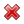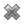# Fight Finance

#### CoursesTagsRandomAllRecentScoresScoreskeithphw $6,011.61 Jade$1,815.80 ZOE HY $860.33 Chu$789.98 royal ne... $750.00 Leehy$713.33 Visitor $650.00 JennyLI$625.61 Visitor $590.00 Visitor$555.33 Visitor $550.00 Visitor$550.00 Visitor $540.00 Visitor$500.00 Yizhou $489.18 Visitor$480.00 Visitor $480.00 Visitor$470.00 Visitor $464.70 Visitor$460.00

A one year European-style call option has a strike price of $4. The option's underlying stock pays no dividends and currently trades at$5. The risk-free interest rate is 10% pa continuously compounded. Use a single step binomial tree to calculate the option price, assuming that the price could rise to $8 $(u = 1.6)$ or fall to$3.125 $(d = 1/1.6)$ in one year. The call option price now is:

A one year European-style put option has a strike price of $4. The option's underlying stock pays no dividends and currently trades at$5. The risk-free interest rate is 10% pa continuously compounded. Use a single step binomial tree to calculate the option price, assuming that the price could rise to $8 $(u = 1.6)$ or fall to$3.125 $(d = 1/1.6)$ in one year. The put option price now is: